# Tutorial for Python ListsThis tutorial goes through how to work with lists in Python, including many of the built-in methods Python makes available for these data structures. Feel free to click on any of the links below to skip to a section of interest.

Background on lists
Referring to list elements by index
Slicing lists
Combining lists
Lists are mutable
Lists support inplace methods

# Background on lists

A list in Python is a container of numbers, strings, or other objects (or mixed). Lists can be created using brackets enclosing whatever elements you want in the list, where each element is separated by a comma.

Below we define a list containing five numbers – 4, 5, 10, 20, and 34.

```
nums = [4, 5, 10, 20, 34]

```

We can also define a list of strings:

```
strings = ["this", "is", "another", "list"]

```

Or – here’s a mixed data type list:

```
["example", "of", "a", 42, 10, "mixed", "data type list", 5]

```

Lists can also contain other lists. These lists are called nested lists. Below we define a list where the last element is just an integer, 100, but the other elements of the list are lists themselves.

```
nested_list = [["inner list", 10], ["second inner list"], 100]

```

## Referring to list elements by index

One property of lists is that each element in the list can be referred to by an index i.e. there is an order to the elements in the list. In other words, there is the concept of the first element, second element, third element, and so on. Since Python is zero-indexed, the 0th element of the list defined above is 4. The 1st element is 5, the 2nd is 10, and so on.

We refer to elements in a list by index using bracket notation like this:

```
nums # returns 4

nums # returns 5

nums # returns 10

```

We can also refer to elements starting from the end of the list using negative indices.

```
nums[-1] # returns 34

nums[-2] # returns 20

nums[-3] # returns 10

```

## Slicing a list

What if want to grab more than one element at a time from a list? For instance, how would we retrieve the first three elements of our nums list?

```
nums[0:3]

nums[:3]

```

Each of the above will return the first three elements of the list nums. The 0:3 means that Python will return the elements in the list indexed 0, 1, and 2 – but not 3. So it will include all the elements up until, but not including the third-indexed element.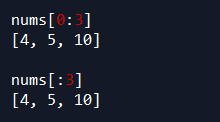Here’s a few more examples:

```
nums[1:4]

nums[1:3]

```

The first example above will retrieve the elements indexed 1, 2, and 3 (but not 4) of nums. The second example retrieves the elements indexed 1 and 2 (but not 3).## How to combine lists

Lists can be combined using the “+” operator.

```
list_1 = [3, 4, 5]
list_2 = [6, 7, 8]

list_3 = list_1 + list_2

list_3

```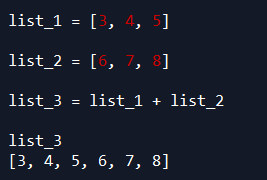We can also chain together multiple concatenation operations:

```
[1, 2, 3] + [4, 5, 6] + ["python", "is", "awesome"] + [["nested list"]]

```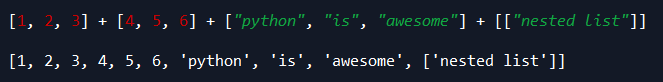## Lists are mutable

Another property of lists is that they are mutable. We’ll see examples of what this means in the below sections. Effectively, though, mutable means that the state of a list can be changed after it has been defined. What does that mean in practice? Mutability means we can change how a list (or some other object in Python for that matter) is defined without formally redefining the list.

Suppose, for example, we wanted to change the 0th element of the strings list above. We can do that by referencing just the 0th element of the list, and setting it equal to some other value.

```
strings = ["this", "is", "another", "list"]

strings = "that"

strings

```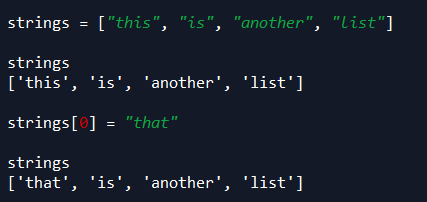The above code is an alternative to the below where we redefine the full list strings. The mutability of lists gives us the ability to shorten the amount of code we write to adjust the values they contain.

```
strings = ["that", "is", "another", "list"]

```

Some of the additional examples below will show how lists can be mutated, or changed, after they have been defined.

## Lists support inplace methods

Lists in Python have several inplace methods. These are methods, or functions, that perform operations on a list with the result being automatically stored back into the same list, thus generally reducing the amount of code that has to be written. If that isn’t clear, we’ll see examples of how this works in several of the sections below.

# Adding elements to a list

## How to append elements in a list

An example of mutability is via appending elements to a list. To append elements to a list, one at at a time, we can use the (aptly-named) append method. This method is also an example of an inplace operation.

```
# append 100 to the end of our list, nums
nums.append(100)

```Below we append 200 to the end of nums, followed by 300.

```
nums.append(200)

nums.append(300)

```Each of these append examples demonstrates how lists are mutable because they each show how the definition of nums can be changed after its initialization without a new initialization. In other words, we could have taken our original list and then redefined that list multiple times to append elements to the end of the list, like this:

```
nums = [4, 5, 10, 20, 34]

nums = [4, 5, 10, 20, 34, 100]

nums = [4, 5, 10, 20, 34, 100, 200]

nums = [4, 5, 10, 20, 34, 100, 200, 300]

```

…But we don’t have to. We can accomplish what the code above does with the append method because of the fact that lists are mutable, and therefore, we can mutate, or change, lists without reinitialization.

Also, because append is inplace, we don’t have to run the last chunk of code above where we’re redefining nums because the method automatically changes nums to have the appended values.

## How to insert elements at specific locations in a list

The append method above appends an element to the end of a list, but what if we want to insert an element at some other specific location in a list? We can perform this task using the insert method.

Taking the previous value of nums as [4, 5, 10, 20, 34, 100, 200, 300], let’s insert the string “test” into the third indexed position of the list.

```
nums.insert(3, "test")

```

The insert method takes two parameters. The first is the index in the list we want to insert some element. The second parameter is the element we want to insert. In this case, we insert the string “test” into the third index of the list, nums.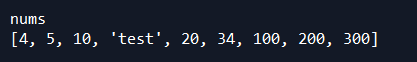Putting an element into the nth position in a list will shift the elements in that position and later to the right. In our case, this means the element in the third position initially will now be in the fourth position, the element in the fourth position shifts to the fifth position, the element in the fifth position shifts to the sixth position, etc.

Below we insert the string “test” again – though this time we put it into the seventh-indexed position.

```
nums.insert(7, "test")

```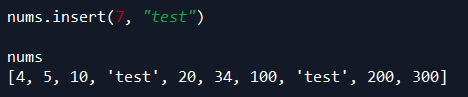## How to append another list of elements to a list

One of my favorite methods for dealing with lists is the extend method because it can append an entire other list of elements at once to your list.

```
nums.extend([1000, 2000, 3000])

```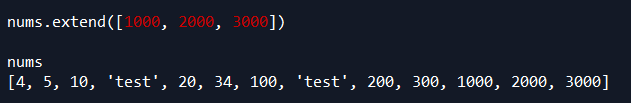The extend method can be viewed as a shortcut for writing the below code:

```
nums = nums + [1000, 2000, 3000]

```

The above code will take the initial list, nums, and append the numbers 1000, 2000, and 3000 to the end of the list – just like the extend method. The difference is that in this case we have to redefine nums to be equal to this concatenation, whereas the extend method is inplace.

# Removing elements from a list

## How to remove elements from a list by specific values

We can remove elements of a list that equal a specific value using the remove method. The remove method will take out the first occurrence of the input value for a given list (and only the first occurrence). For instance, let’s say we want to get rid of the string “test” we inserted into nums in an earlier section.

```
# remove first occurrence of "test"
nums.remove("test")

# remove next occurrence of "test"
nums.remove("test")

```

Each time we run nums.remove(“test”), Python will remove the first found occurrence of the string “test” in the list. Thus, running the first line above will remove “test” from the third-indexed position in nums. Running the second line will remove the next (and in this case, only other) occurrence of “test” in the list.## How to remove an element from a specific index

Elements can also be removed from a list based off index. For example, if we want to remove the element in the third-indexed position from a list, we could do this:

```
# remove element in the third-indexed position
nums.pop(3)

# or remove the element in the fifth-indexed position
nums.pop(5)

```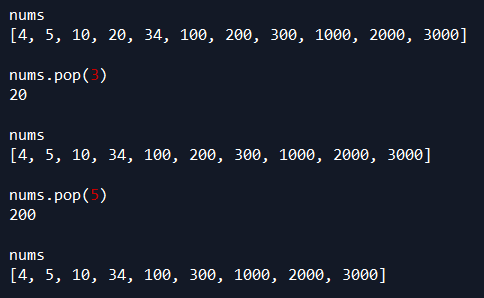Notice from the snapshot above that the pop method returns the value of the element that is removed. Hence, when used above, it returns 20 and then 200, respectively.

Now, we can insert those numbers back into our list:

```
nums.insert(5, 200)

nums.insert(3, 20)

```## How to remove all elements of a list

To clear out all elements of a list we can use the clear method:

```
nums.clear()

```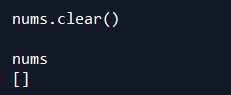# Other built-in list operations

## Filtering lists

Lists can be filtered using the filter function. The filter function works by taking a function and a list as input. The input function applies a logical condition that returns True or False (i.e. Boolean) for each element in the list. If the function returns True, the output object will retain that list element.

The actual object returned by filter is not a list (as of Python >= 3.0) – but instead, is called a filter object. To convert this to a list, we just need to wrap the list function around the filter object.

```
a = [10, 20, 30, 40]

filter(lambda num: num < 30, a)

```
```
list(filter(lambda num: num < 30, a))

```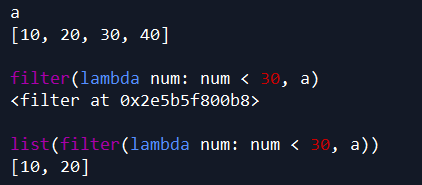## How to reverse the elements in a list

#### Option 1)

There’s a few different ways to reverse the elements in a list. One way is using the inplace method called reverse, like so:

```
nums.reverse()

```

This will reverse the elements of our list, nums, while storing the results of that reversal back in nums. So if we run the below line of code, we can see now that nums contains its original elements in reverse.

```
nums

```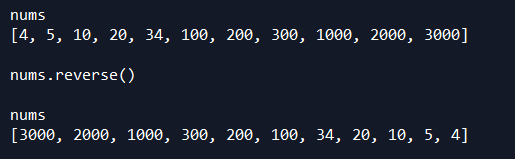#### Option 2)

Another way of reversing a list is using bracket notation, like this:

```
nums[::-1]

```

Doing the above will reverse the elements of nums, but won’t store the results back into nums unless we tell it to, like this:

```
nums = nums[::-1]

```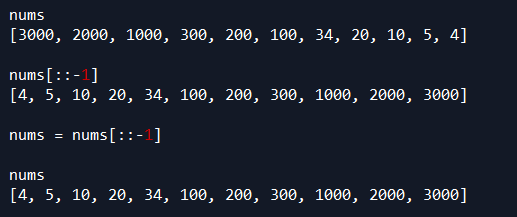## How to count the occurrence of an element in a list

To count how many times an element occurs in a list, we can use the count method, like this (using a different list this time):

```
x = [10, 10, 30, 20, 50, 50, 30, 30, 30]

x.count(30)

```

Here, running x.count(30) will return 4 because 30 occurs four times in x.Likewise, if we want to count how many times 50 appears, or 10 appears, we would do this:

```
x.count(50)

x.count(10)

```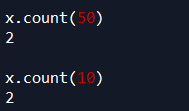If we try to use the count method for an element that doesn’t occur in the list, Python will return zero:

```
x.count(100)

```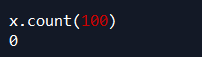## How to copy a list

Lists can be copied using the copy method.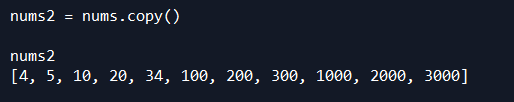## How to sort a list

Sorting a list can be done using the sort method like this:

```
# define a new list
new_list = [10, 4, 17, 21, 8, 12, 2]

# sort list
new_list.sort()

# see results stored in new_list
new_list

```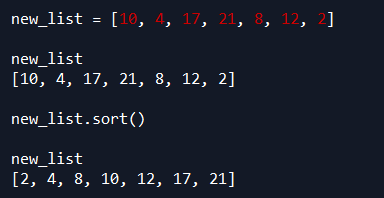You can also sort a list using the sorted function:

```
sorted_list = sorted(new_list)

```

## How to get the length of a list

Getting the length of a list can be done in a couple of different ways. The first, more commonly seen way, is using Python’s built-in len function.

```
a = [4, 5, 6]
len(a) # returns 3

b = [10, 20, 30, 40, 50]
len(b) # returns 5

```

You can also get the length of a list using the __len__ method. Note the double underscore on each side of len.

```

a = [4, 5, 6]
a.__len__() # returns 3

b = [10, 20, 30, 40, 50]
b.__len__() # returns 5

```

## How to get the index where an element first occurs

Figuring out where in a list an element first occurs can be done using the index method.

```
strings = ["this", "is", "another", "list"]

strings.index("another") # returns 2

strings.index("list") # returns 3

```